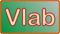onlineoverland:   Overland flow using the diffusion wave method --- One bookReferences Ponce, V. M. 1986. Diffusion wave modeling of catchment dynamics. Journal of Hydraulic Engineering, 112(8), August, 716-727. Ponce, V. M. 1989. Engineering Hydrology: Principles and Practices, Prentice Hall, Englewood Cliffs, New Jersey, 322-325.

 DESCRIPTION: [Sample Input] [Main Page] [Rationale]

This program simulates catchment dynamics using the diffusion wave model developed by Ponce (1986). The program is a numerical model of overland flow using an open-book schematization.

A significant feature of the model is its grid independence, allowing it to calculate the same runoff hydrograph, regardless of the grid specification [provided the problem is well defined]. The only grid input is the time interval Δt. Upon execution, the program adjusts the value of the space interval Δx in planes and channel to assure grid independence and preserve modeling accuracy.

The following input values are described here in detail.

• Units:  The program can use either SI (Metric) units of U.S. Customary units, selectable on input. The applicable units are displayed to the left of the input box.

• The rainfall depth P is specified in cm or in., depending on the units selected. The input value is limited to a value greater than zero (0).

• The runoff curve number CN is limited to a value greater than 20.

• The rainfall duration tr is specified in hours. The input value is limited to a value greater than zero (0).

• The rainfall distribution is specified in three parts: (1) the number of points Np in the cumulative rainfall distribution, (2) the dimensionless cumulative time T*, and (3) the dimensionless cumulative depth D*. For instance, for a uniform distribution, the number of points Np is 2; the dimensionless cumulative time T* is entered as 0.,1.; likewise, the the dimensionless cumulative depth D* is entered as 0.,1.

• The total simulation time Tt is specified in hours. The input value is limited to a value greater than zero (0).

• The number of time intervals NΔt is limited to an integer greater than zero (0).

• The number of time intervals for printing Npr is an integer greater than zero (0). A normal value is Npr = 1, that is, output is reported every time interval. For Npr = 2, output is reported every two time intervals.

• The fraction used to estimate reference discharge Fr is specified in the range 0 ≤ Fr ≤ 1. Normally, the value of Fr = 0.5; leave the input box blank to specify the default value Fr = 0.5.

• The overland flow area A is specified in hectares or acres, depending on the units selected. The allowable range is 0 ≤ A ≤ 2500 in SI (metric) units; 0 ≤ A ≤ 2500 in U.S. Customary units.

• The fraction of area in the left plane Fr is specified in the range 0 < Fr ≤ 1. Enter Fr = 1 to specify only one (left) plane.

• The slope of the left plane Slp, limited in the range 0.000001 ≤ Slp ≤ 0.2.

• The Manning coefficient for the left plane nlp, limited in the range 0.008 ≤ nlp ≤ 1.

• The rating exponent for the right plane βlp, limited in the range 1.5 ≤ nlp ≤ 3. To specify default value of β = 2, enter the value -1 in the input box.

• The slope of the right plane Slp, limited in the range 0.000001 ≤ Srp ≤ 0.2.

• The Manning coefficient for the right plane nlp, limited in the range 0.008 ≤ nrp ≤ 1.

• The rating exponent for the right plane βlp, limited in the range 1.5 ≤ nrp ≤ 3. To specify default value of β = 2, enter the value -1 in the input box. Leave input box blank to specify right plane to exactly mirror left plane for Flp = 0.5 only.

• The length of the channel Lch is specified in m or ft, depending on the units selected. The input value is limited to a value greater than zero (0).

• The slope of the channel Sch, limited in the range 0.000001 ≤ Slp ≤ 0.2.

• The Manning coefficient of the channel nch, limited in the range 0.008 ≤ nch ≤ 0.250.

• The bottom width of the channel Bch is specified in m or ft, depending on the units selected. The input value is limited to a value greater than zero (0).

• The design depth of the channel ych is specified in m or ft, depending on the units selected. The input value is limited to a value greater than zero (0).

• The side slope of the channel z [z H : 1 V] is limited in the range 0 ≤ z ≤ 10.

Program ONLINEOVERLAND will trigger one of thirty-two (32) fatal error messages if any input value is out of range. Additionally, the program will trigger a warning error message (Error 33) if the [calculated] maximum channel flow depth is greater than the design depth of the channel.

The current version  can only handle one book. The capability to handle several books is under development at this time.

Thank you for running   onlineoverland.php